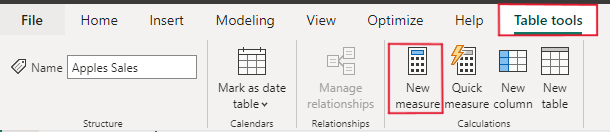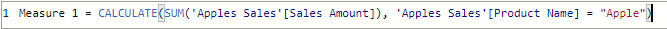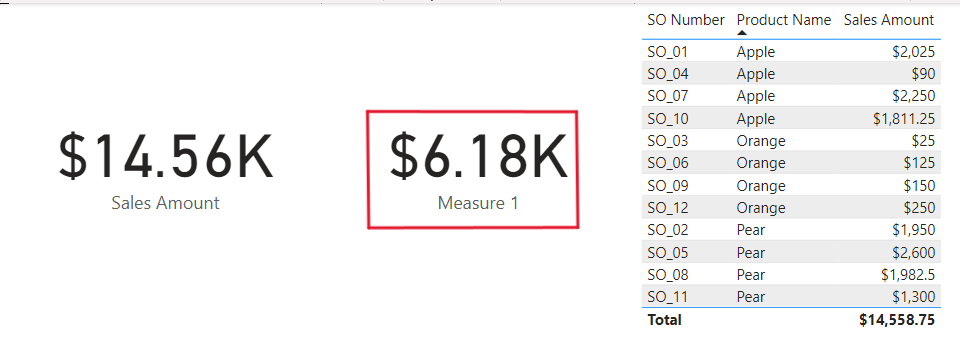Email : info@epmstrategy.com | Call Us Now : 479-321-3977
:: Home :: Sitemap ::

# Power BI – DAX Calculate Function

BY: David Rohlfs

Introduction

When you work in Microsoft Power BI, there is a constant need for growth in your DAX and M Code knowledge. But it can be hard to know where to start. For DAX, one of the best places to start is with the Calculate function. The calculate function can do an aggregation on a field and filter a table by your specifications.

In this blog we are going to go through when you should use a calculate function and a short walkthrough demonstrating how to use this function.

When to use it

The beauty of the calculate function is that it satisfies most of the needs for a DAX measure. While this is not the only reason you will need a measure, most of the time you are trying to find a simple calculation of a field with a filter or two. And when you need this the Calculate function does it very easily. What the calculate function does is an aggregation and a filter. Here is what the syntax looks like:

Calculate( <Aggregation([Column])>, <Filter>)

While the calculate function is great at doing simple aggregations on a field, what are some complex uses for it?

This is something that is smart to ask when you are looking into DAX functions because it shows the full capabilities of the function. What I like to use the calculate function for besides normal measures is to find important values in larger calculations. This often comes into the use of Variables in DAX.

Think of this example: I need to have a variable that contains the number of days between my earliest and latest order for the month of May. I will write a variable that looks like this:

Running Days in May = Calculate( Count( DatesBetween( ‘SalesTable’, Min( ‘SalesTable’[OrderDate]), Max( ‘SalesTable’[OrderDate]),))), Month(‘SalesTable’[OrderDate]) = “May” && Year(‘SalesTable’[OrderDate]) = “2023”)

Then you can place a variable like this in a larger equation that needs this value.

While there are a lot of uses for the calculate function, the calculate function really shines when you get into simple needs of aggregations within DAX. Using a calculate function as a portion of an equation opens real possibilities to complex equations that have a necessity for simple parts of a complex whole.

Walkthrough

In this walkthrough we are going to demonstrate the calculate function by using it to create a card. I want to stay simple with this so that you can see how just the calculate function works whether it’s on its own or inside of a large complex equation.

To start we are going to need to create a measure. You can do this by clicking on your table in the fields list and select New Measure in the Table Tools. This will bring up this equation bar underneath the ribbon.Next, we can write out our equation inside of the formula bar. I am just going to create a sum of all the Apple Sales.Now we can place our measure in a card, and here is what this function’s end value was.Conclusion

While it is easy to learn the basics of the calculate function, there are a lot of complex uses for it. You can easily start to create large equations inside of the DAX column or measure that needs the calculate function to help simplify the syntax. If this blog was interesting to you, you might like to read through some of EPM Strategy’s other blogs that focus on DAX and DAX functions linked below.

Power BI Understanding the Basics of Using Variables in DAX : :: Welcome To EPM Strategy ::

DAX If Statement

Power BI Conditional Formatting with DAX : :: Welcome To EPM Strategy ::

DAX ABS Function

Power BI Round Function in DAX : :: Welcome To EPM Strategy ::

Power BI DAX HasOneValue : :: Welcome To EPM Strategy ::

The Differences Between Creating a Report on Power BI Service VS. Desktop : :: Welcome To EPM Strategy ::